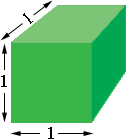SEARCH HOMEMath Central Quandaries & QueriesQuestion from wyatt, a student: what is a cubic inch?Hi Wyatt,

A cubic inch is a unit of volume. It is the volume of a cube that is 1 inch long by 1 inch wide by 1 inch tall.Suppose you have a box that is 4 inches long by 3 inches wide by 2 inches high and you have a collection of 1 inch cubes as in the diagram above. On the bottom of the box you could place 3 rows of 4 blocks each to form a layer of $3 \times 4 = 12$ blocks. The box is 2 inches high so you can place another layer of 12 blocks on top of the previous layer to fill the box with a volume of $3 \times 4 \times 2 = 12$ cubic inches.

I hope this helps,
PennyMath Central is supported by the University of Regina and The Pacific Institute for the Mathematical Sciences.